Win up to 100% scholarship on Aakash BYJU'S JEE/NEET courses with ABNAT Win up to 100% scholarship on Aakash BYJU'S JEE/NEET courses with ABNAT

# JEE Main 2022 July 26 – Shift 2 Chemistry Question Paper with Solutions

The JEE Main 2022 July 26 – Shift 2 Chemistry Question Paper with Solutions is given on this page. These solutions are prepared by subject experts at BYJU’S. Students can use the JEE Main 2022 answer keys to calculate their scores in the JEE Main 2022 exams. They are recommended to practise and learn the JEE Main 2022 question paper with solutions so that they can improve their performance in the upcoming exams.

## JEE Main 2022 26th July Shift 2 Chemistry Question Paper and Solutions

SECTION – A

Multiple Choice Questions: This section contains 20 multiple choice questions. Each question has 4 choices (1), (2), (3) and (4), out of which ONLY ONE is correct.

1. Haemoglobin contains 0.34% of iron by mass. The number of Fe atoms in 3.3 g of haemoglobin is

(Given: Atomic mass of Fe is 56 u, NA = 6.022 × 1023 mol–1)

(A) 1.21 × 105

(B) 12.0 × 1016

(C) 1.21 × 1020

(D) 3.4 × 1022

Sol. According to the question,

100 g of haemoglobin contains 0.34 g of iron
3.3 g of haemoglobin contains

$$\begin{array}{l} \frac{0.34}{100}\times3.3\text{ g}\end{array}$$
of iron moles of Fe
$$\begin{array}{l} =\frac{0.34\times3.3}{100\times56}=\frac{N}{N_A}\end{array}$$
$$\begin{array}{l} N=\frac{0.34\times3.3\times6.022\times10^{23}}{100\times56}= 1.21 \times 10^{20} \end{array}$$

2. Arrange the following in increasing order of their covalent character.

A. CaF2

B. CaCl2

C. CaBr2

D. CaI2

Choose the correct answer from the option given below.

(A) B < A < C < D

(B) A < B < C < D

(C) A < B < D < C

(D) A < C < B < D

Sol. From Fajan’s rule, for a given metal ion, as the size of anion increases, polarizability of anion increases and hence covalent character of the given ionic compound increases.

Hence, the increasing order of covalent characters is:

CaF2< CaCl2< CaBr2< CaI2

3. Class XII students were asked to prepare one litre of buffer solution of pH 8.26 by their Chemistry teacher. The amount of ammonium chloride to be dissolved by the student in 0.2 M ammonia solution to make one litre of the buffer is

(Given : pKb (NH3) = 4.74, Molar mass of NH3 = 17 g mol–1, Molar mass of NH4Cl = 53.5 g mol–1)

(A) 53.5 g

(B) 72.3 g

(C) 107.0 g

(D) 126.0 g

Sol. For basic Buffer,

$$\begin{array}{l} pOH = pK_b+\text{log}\frac{\left[\text{salt}\right]}{\left[\text{Base}\right]} \end{array}$$
$$\begin{array}{l} pOH = 14 – 8.26 = 5.74\\5.74=4.74+log\frac{[NH_4Cl]}{0.2}\end{array}$$
$$\begin{array}{l} \left[NH_4Cl\right]=2\text{ M}\end{array}$$

Moles of NH4Cl = 2 × 1 = 2 moles

Weight of NH4Cl = 2 × 53.5 = 107 g

4. At 30ºC, the half life for the decomposition of AB2 is 200 s and is independent of the initial concentration of AB2. The time required for 80% of the AB2 to decompose is

(Given : log 2 = 0.30, log 3 = 0.48)

(A) 200 s

(B) 323 s

(C) 467 s

(D) 532 s

Sol. Since, half life is independent of the initial concentration of AB2. Hence, reaction is “First Order”.

$$\begin{array}{l} k=\frac{2.303\text{ log} 2}{t_{1/2}}\end{array}$$
$$\begin{array}{l} \frac{2.303\text{ log}2 }{t_{1/2}}=\frac{2.303}{t}\text{ log}\frac{100}{\left(100-80\right)}\end{array}$$
$$\begin{array}{l} \frac{2.303\times0.3}{200}=\frac{2.303}{t}\text{log}5 \\t = 467 ~\text{s}\end{array}$$

5. Given below are two statements: one is labelled as Assertion A and other is labelled as Reason R.

Assertion A : Finest gold is red in colour, as the size of the particles increases, it appears purple then blue and finally gold.

Reason R : The colour of the colloidal solution depends on the wavelength of light scattered by the dispersed particles.

In the light of the above statements, choose the most appropriate answer from the options given below.

(A) Both A and R are true and R is the correct explanation of A

(B) Both A and R are true but R is NOT the correct explanation of A

(C) A is true but R is false

(D) A is false but R is true

Sol. Finest gold sol is red in colour; as the size of particles increases, it appears purple, then blue and finally golden.

The colour of colloidal solution depends on the wavelength of light scattered by the dispersed particles. The wavelength of light further depends on size and nature of the particles.

Hence, Both A and R are true and R is the correct explanation of A.

6. The metal that has very low melting point and its periodic position is closer to a metalloid is

(A) Al

(B) Ga

(C) Se

(D) ln

Sol Among the given elements, Gallium has the lowest melting point. Gallium is also close to a metalloid.

7. The metal that is not extracted from its sulfide ore is

(A) Aluminium

(B) Iron

(D) Zinc

Sol Aluminium is not extracted from sulphide ore. It is usually extracted from bauxite ore, leaching of bauxite ore is done followed by electrolytic reduction.

8. The products obtained from a reaction of hydrogen peroxide and acidified potassium permanganate are

(A) Mn4+, H2O only

(B) Mn2+, H2O only

(C) Mn4+, H2O, O2 only

(D) Mn2+, H2O, O2 only

Sol

$$\begin{array}{l} 2MnO_4^-+6H^++5H_2O_2\xrightarrow[~~~~~]{}2Mn^{2+}+8H_2O +5O_2\end{array}$$

This reaction shows reducing action of H2O2 in acidic medium.

The products formed are Mn2+, H2O and O2.

9. Given below are two statements: one is labelled as Assertion A and the other is labelled as Reason R.

Assertion A : LiF is sparingly soluble in water.

Reason R : The ionic radius of Li+ ion is smallest among its group members, hence has least hydration enthalpy.

In the light of the above statements, choose the most appropriate answer from the options given below.

(A) Both A and R are true and R is the correct explanation of A

(B) Both A and R are true but R is NOT the correct explanation of A

(C) A is true but R is false.

(D) A is false but R is true.

Sol LiF is sparingly soluble in water.

The low solubility of LiF in water is due to its high lattice enthalpy (Since Li+ and F are small in size). Also, due to small size of Li+, its hydration enthalpy is high.

Hence, Assertion is true, but Reason is false.

10. Given below are two statements: one is labelled as Assertion A and the other is labelled as Reason R.

Assertion A: Boric acid is a weak acid

Reason R: Boric acid is not able to release H+ ion on its own. It receives OH ion from water and releases H+ ion.

In the light of the above statements, choose the most appropriate answer from the options given below.

(A) Both A and R are correct and R is the correct explanation of A.

(B) Both A and R are correct but R is NOT the correct explanation of A

(C) A is correct but R is not correct

(D) A is not correct but R is correct

Sol Boric acid is a weak acid

$$\begin{array}{l} H_3BO_3+H_2O\rightleftharpoons \left[B\left(OH\right)_4\right]^-+H^{\bigoplus} \end{array}$$

Boric acid is not able to release H+ ion on its own. It receives OH ion from water and releases H+ ion as shown in the above reaction.

Hence, Both A and R are correct and R is the correct explanation of A.

11. The metal complex that is diamagnetic is (Atomic number : Fe, 26; Cu, 29)

(A) K3[Cu(CN)4]

(B) K2[Cu(CN)4]

(C) K3[Fe(CN)4]

(D) K4[FeCl6]

Sol. ⇒ K3[Cu(CN)4] is diamagnetic

Cu(I) ⇒ d10 configuration ⇒ No unpaired electrons.

⇒ K2[Cu(CN)4], K3[Fe(CN)4] and K4[FeCl6] are paramagnetic in nature.

12. Match List I with List II.

List I

Pollutant

List II

Source

A. Microorganisms I. Strip mining
B. Plant nutrients II. Domestic sewage
C. Toxic heavy metals III. Chemical fertilizer
D. Sediment IV. Chemical factory

Choose the correct answer from the options given below:

(A) A-II, B-III, C-IV, D-I

(B) A-II, B-I, C-IV, D-III

(C) A-I, B-IV, C-II, D-III

(D) A-I, B-IV, C-III, D-II

Sol. Pollutant Source

Microorganisms → Domestic sewage

Plant nutrients → Chemical fertilizers

Toxic heavy metals → Chemical factory

Sediment → Strip mining

13. The correct decreasing order of priority of functional groups in naming an organic compound as per IUPAC system of nomenclature is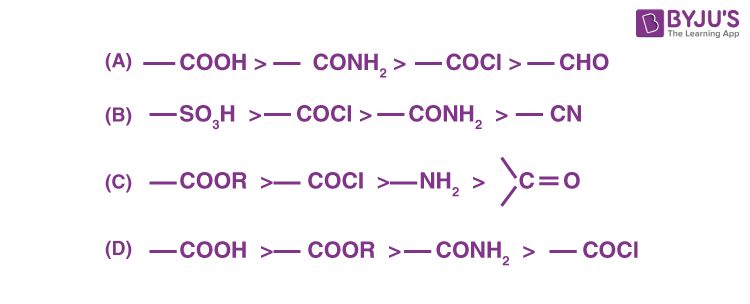Sol. The order of decreasing priority for functional group is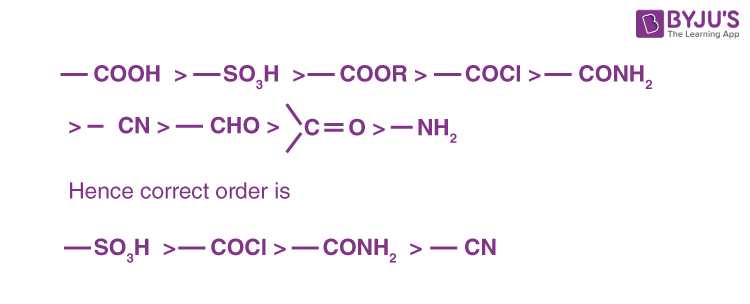14. Which of the following is not an example of benzenoid compound?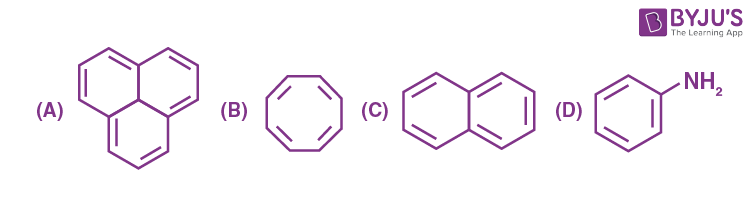Sol.are not benzenoid compounds, since benzenoid compound contains benzene ring.

15. Hydrolysis of which compound will give carbolic acid?

(A) Cumene

(B) Benzenediazonium chloride

(C) Benzal chloride

(D) Ethylene glycol ketal

Sol. Phenol, is known as Carbolic acid.

Diazonium salt are hydrolysed to phonols.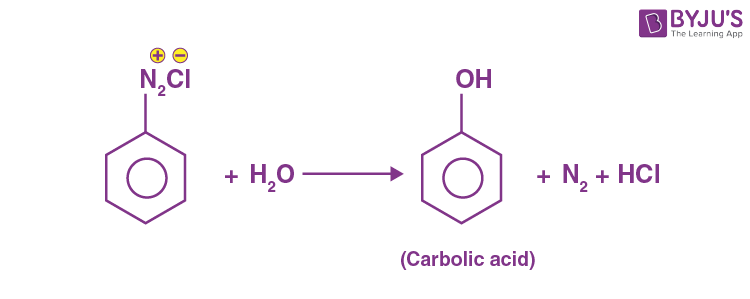Benzal chloride on hydrolysis gives benzaldehyde.

16.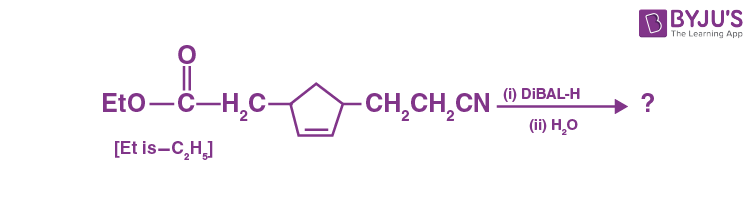Consider the above reaction and predict the major product.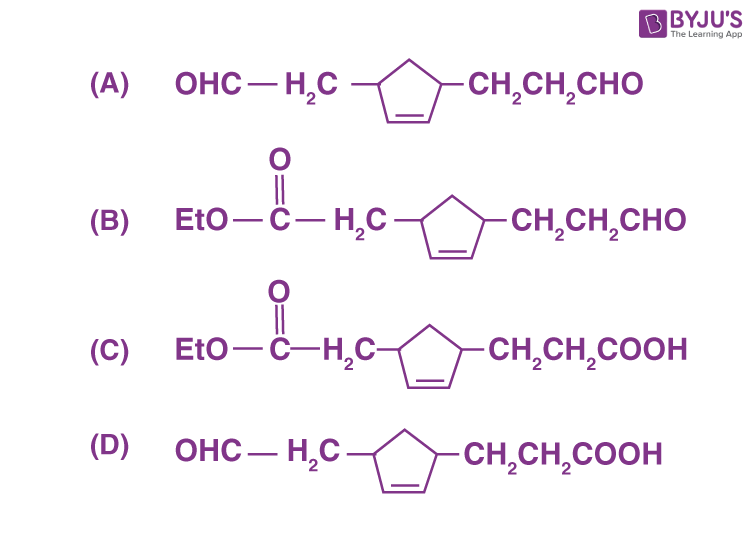Sol. DIBAL-H reduces both the cyanides and esters to aldehydes.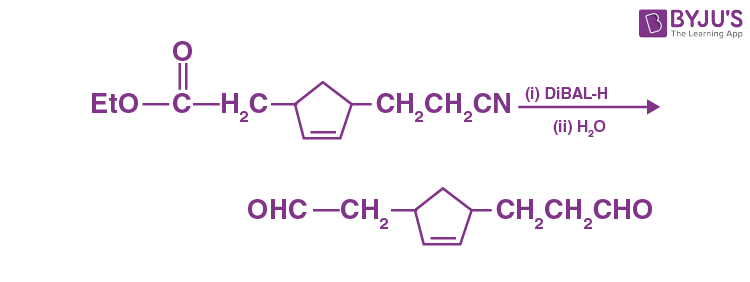17. The correct sequential order of the reagents for the given reaction is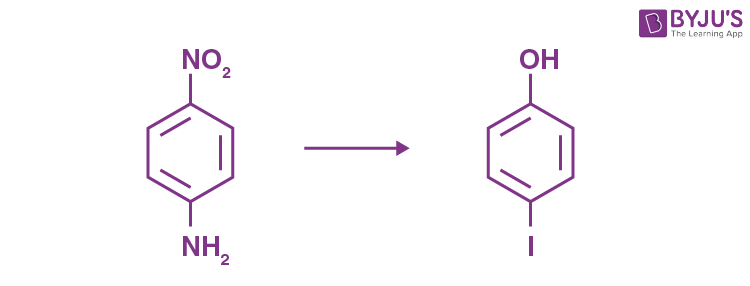(A) HNO2, Fe/H+, HNO2, KI, H2O/H+

(B) HNO2, KI, Fe/H+, HNO2, H2O/warm

(C) HNO2, KI, HNO2, Fe/H+, H2O/H+

(D) HNO2, Fe/H+, KI, HNO2, H2O/warm

Sol.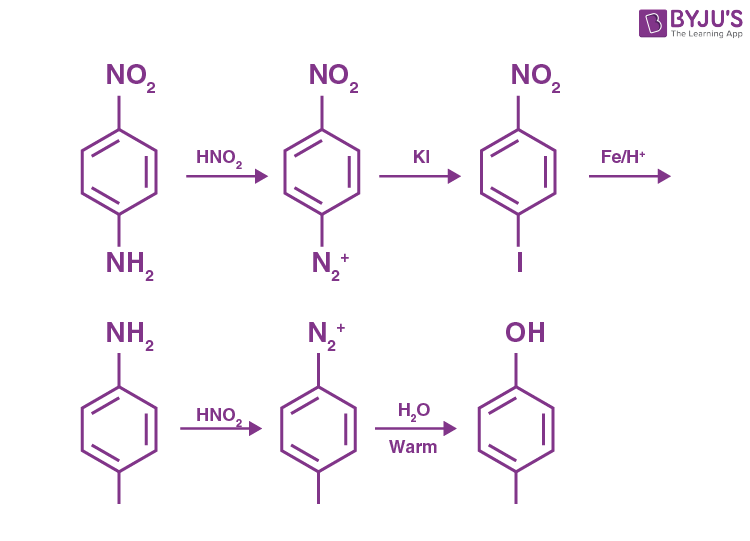18. Vulcanization of rubber is carried out by heating a mixture of

(A) isoprene and styrene

(B) neoprene and sulphur

(C) isoprene and sulphur

(D) neoprene and styrene

Sol. When a mixture of isoprene and sulphur is heated, isoprene gets polymerised to natural rubber and then vulcanization of natural rubber with sulphur takes place.

19. Animal starch is the other name of

(A) amylose

(B) maltose

(C) glycogen

(D) amylopectin

Sol. Animal starch is the other name of glycogen because its structure is similar to amylopectin.

20. Given below are two statements: One is labelled as Assertion A and the other is labelled as Reason R.

Assertion A: Phenolphthalein is a pH dependent indicator, remains colourless in acidic solution and gives pink colour in basic medium.

Reason R: Phenolphthalein is a weak acid. It doesn’t dissociate in basic medium.

In the light of the above statements, choose the most appropriate answer from the options given below.

(A) Both A and R are true and R is the correct explanation of A

(B) Both A and R are true but R is NOT the correct explanation of A

(C) A is true but R is false

(D) A is false but R is true

Sol. Phenolphthalein is a pH dependent indicator. It is a weak acid which is colourless in the acidic solution but gives pink colour in basic medium. The pink colour is due to its conjugate form. Therefore, assertion (A) is true but Reason (R) is false.

SECTION – B

Numerical Value Type Questions: This section contains 10 questions. In Section B, attempt any five questions out of 10. The answer to each question is a NUMERICAL VALUE. For each question, enter the correct numerical value (in decimal notation, truncated/rounded-off to the second decimal place; e.g. 06.25, 07.00, –00.33, –00.30, 30.27, –27.30) using the mouse and the on-screen virtual numeric keypad in the place designated to enter the answer.

1. A 10 g mixture of hydrogen and helium is contained in a vessel of capacity 0.0125 m3 at 6 bar and 27°C. The mass of helium in the mixture is _______ g. (nearest integer)

Given : R = 8.3 J K–1 mol–1

(Atomic masses of H and He are 1 u and 4 u, respectively)

Sol. Number of moles of mixture of H2 and He

$$\begin{array}{l} =\frac{PV}{RT}\end{array}$$
$$\begin{array}{l} =\frac{6\times10^5\times0.0125}{8.3\times300}=3\end{array}$$

Let the mass of He in 10 g mixture be x g

$$\begin{array}{l} \therefore\ \frac{x}{4}+\frac{10-x}{2}=3\end{array}$$

On solving x = 8 g

∴ Mass of He in the mixture = 8 g

2.

$$\begin{array}{l}\text{Consider an imaginary ion}\ _{22}^{48}X^{3-} .\end{array}$$
The nucleus contains ‘a’% more neutrons than the number of electrons in the ion. The value of ‘a’ is ________. [nearest integer]

Sol.

$$\begin{array}{l}\text{Number of electrons in}\ _{22}^{48}X^{3-}\ \text{is}\ 25.\end{array}$$

Number of neutrons = 48 – 22 = 26.

Percentage increase in the number of neutrons over electrons

$$\begin{array}{l}= \left(\frac{26 – 25}{25}\right)100 = 4 \% \\ \therefore a = 4\end{array}$$

3. For the reaction

H2F2(g) → H2(g) + F2(g)

ΔU = –59.6 kJ mol–1 at 27°C.

The enthalpy change for the above reaction is (–) _______ kJ mol–1 [nearest integer]

Given: R = 8.314 J K–1 mol–1.

Sol.

$$\begin{array}{l}H_2F_2(g)\rightarrow H_2(g) + F_2(g)\\ \Delta U = -59.6 ~\text{kJ mol}^{-1} at ~27~^\circ C\end{array}$$
$$\begin{array}{l}\Delta H = \Delta U + \Delta n_gRT \end{array}$$
$$\begin{array}{l} =-59.6+\frac{1\times8.314\times300}{1000}\end{array}$$
$$\begin{array}{l}= -57.10~ \text{kJ mol}^{-1}\end{array}$$

4. The elevation in boiling point for 1 molal solution of non-volatile solute A is 3 K. The depression in freezing point for 2 molal solution of A in the same solvent is 6 K. The ratio of Kb and Kf i.e., Kb/Kf is 1 : X. The value of X is [nearest integer]

Sol. Molality of a solution of non volatile solute (A) = 1

Elevation in boiling point is given by

ΔTb = Kb m

3 = Kb × 1 … (1)

Molality of (A) in the same solvent = 2

Depression in freezing point is given by

ΔTf = Kf m

6 = Kf × 2 … (2)

Dividing (1) by (2)

$$\begin{array}{l} \frac{K_b}{K_f}=\frac{1}{X}=\frac{1}{1} \end{array}$$
$$\begin{array}{l} \therefore X = 1\end{array}$$

5. 20 mL of 0.02 M hypo solution is used for the titration of 10 mL of copper sulphate solution, in the presence of excess of KI using starch as an indicator. The molarity of Cu2+ is found to be ________ × 10–2 M. [nearest integer]

$$\begin{array}{l}Given : 2 Cu^{2+} + 4 I^- \rightarrow Cu_2I_2 + I_2\end{array}$$
$$\begin{array}{l} I_2+2S_2O_3^{2-}\rightarrow 2I^-+S_4O_6^{2-} \end{array}$$

Sol.

$$\begin{array}{l}2Cu^{2+} + 4I^- \rightarrow Cu_2I_2 + I_2\end{array}$$
$$\begin{array}{l} I_2+S_2O_3^{2-}\rightarrow 2I^-+S_4O_6^{2-} \end{array}$$

Milliequivalents of hypo solution = 0.02 × 20 = 0.4

Milliequivalents of Cu2+ in 10 mL solution =

Milliequivalents of I2 = Milliequivalents of hypo

= 0.4

Millimoles of Cu2+ ions in 10 mL = 0.4

$$\begin{array}{l}\text{Molarity of}\ Cu^{2+}\ \text{ions}=\frac{0.4}{10}= 0.04\text{ M}\end{array}$$
$$\begin{array}{l}= 4 \times 10^{-2}~ \text{M}\end{array}$$

6. The number of non-ionisable protons present in the product B obtained from the following reactions is_____.

$$\begin{array}{l} C_2H_5OH+PCl_3\rightarrow C_2H_5Cl+A\\A+PCl_3\rightarrow B \end{array}$$

Sol. PCl3 + C2H5OH → C2H5Cl + H3PO3

(A)

H3PO3 + PCl3→ H4P2O5

(A) (B)

Structure of H4P2O5Total 2 non-ionizable protons are present.

7. The spin-only magnetic moment value of the compound with strongest oxidizing ability among MnF4, MnF3 and MnF2 is____ B.M. [nearest integer]

Sol. MnF3 has the strongest oxidising ability

$$\begin{array}{l} \begin{bmatrix}E_{\text{Mn}^{3+}/\text{Mn}^{2+}}^\circ\simeq 1.57\ V \\\text{\&}\ E_{\text{Mn}^{4+}/\text{Mn}^{2+}}^\circ\simeq 1.2\ V\end{bmatrix}\end{array}$$
So, spin only magnetic moment

$$\begin{array}{l} =\sqrt{4\left(4+2\right)}=\sqrt{24}\text{ B.M.}\end{array}$$
$$\begin{array}{l} \simeq 5 \end{array}$$

8. Total number of isomers (including stereoisomers) obtained on monochlorination of methylcyclohexane is____.

Sol. Compounds formed on mono-chlorination of methylcyclohexane are :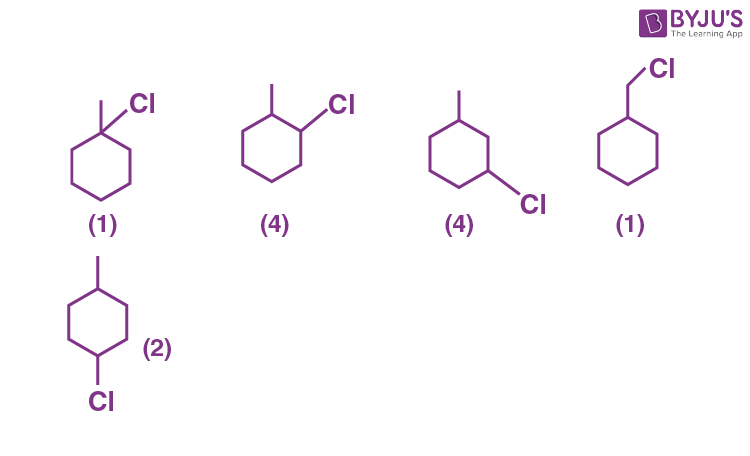∴ Total mono-chlorinated products formed = 12

9. A 100 mL solution of CH3CH2MgBr on treatment with methanol produces 2.24 mL of a gas at STP. The weight of gas produced is_____ mg. [nearest integer]

Sol.

$$\begin{array}{l} CH_3-CH_2-MgBr+CH_3OH\rightarrow CH_3-CH_3+MgBr\left(OCH_3\right)\end{array}$$

As 2.24 ml is formed at STP.

Number of moles of ethane gas produced

$$\begin{array}{l} =\frac{2.24X}{22.4} \\= 10^{-4}~\text{ml}\end{array}$$

Mass of ethane produced = 10–4 × 30 = 3 × 10–3 = 3 mg

10. How many of the following drugs is/are examples(s) of broad-spectrum antibiotics?

Ofloxacin, Penicillin G, Terpineol, Salvarsan.

Sol. Ofloxacin is the only broad spectrum antibiotic given in the question

Penicillin – G is a narrow spectrum antibiotic.

Salvarsan is mainly active against spirochete, a bacteria that causes syphilis

Terpineol is an antiseptic.

### Download PDF of JEE Main 2022 July 26 Shift 2 Chemistry Paper & Solutions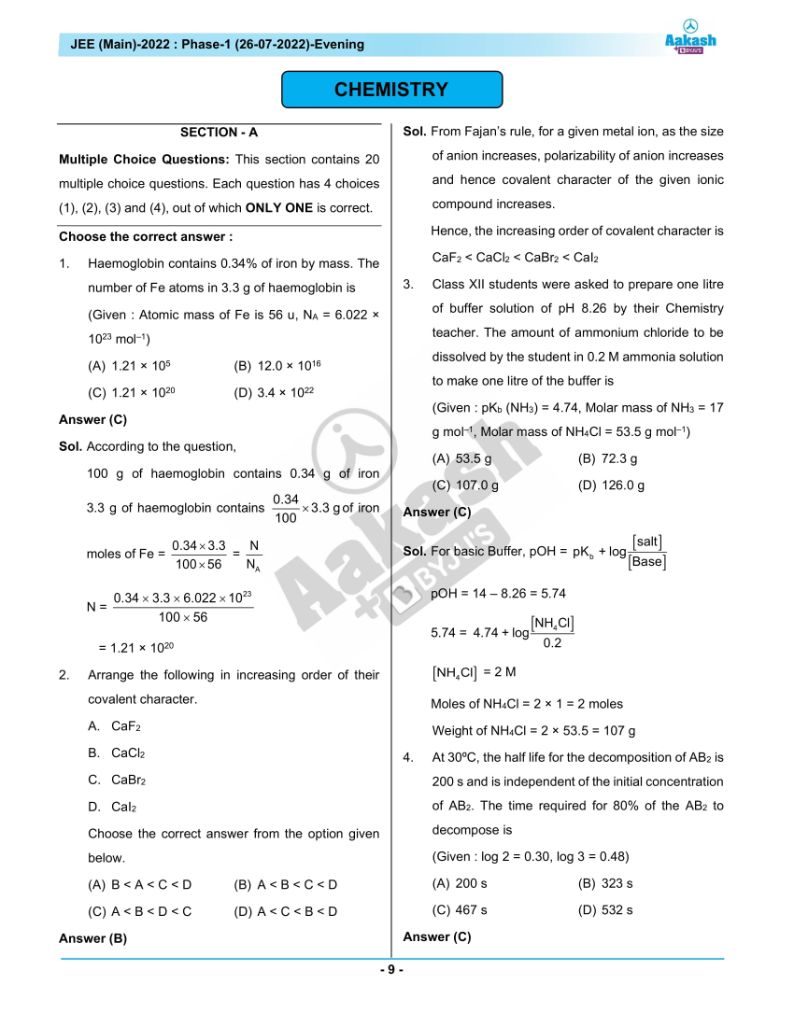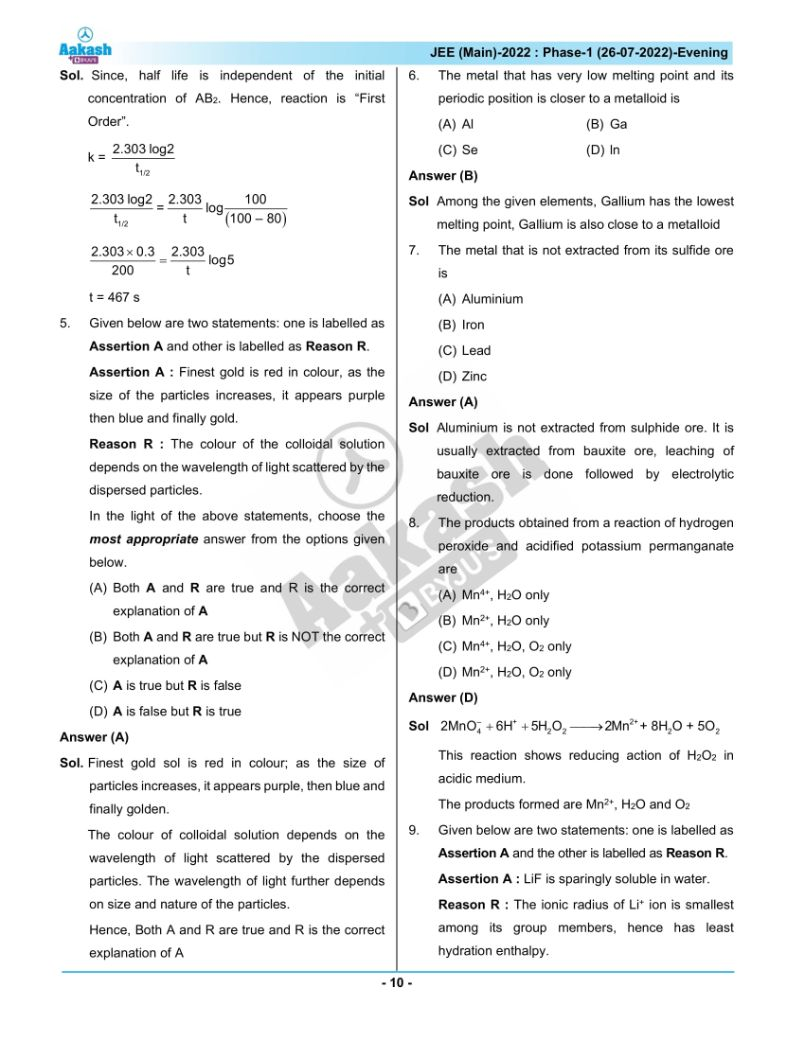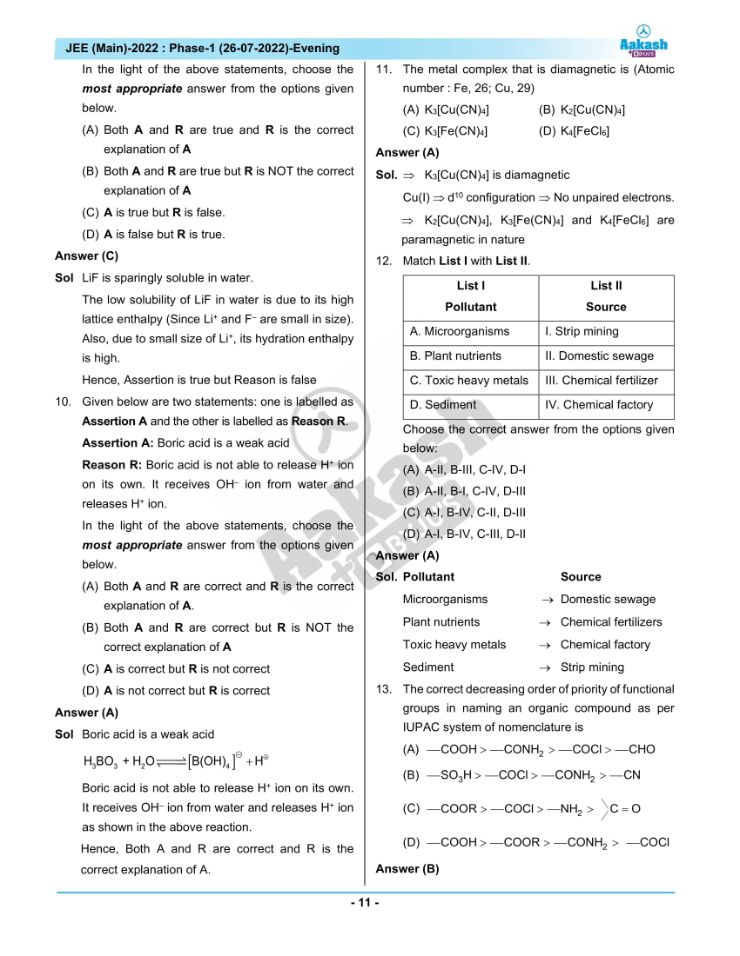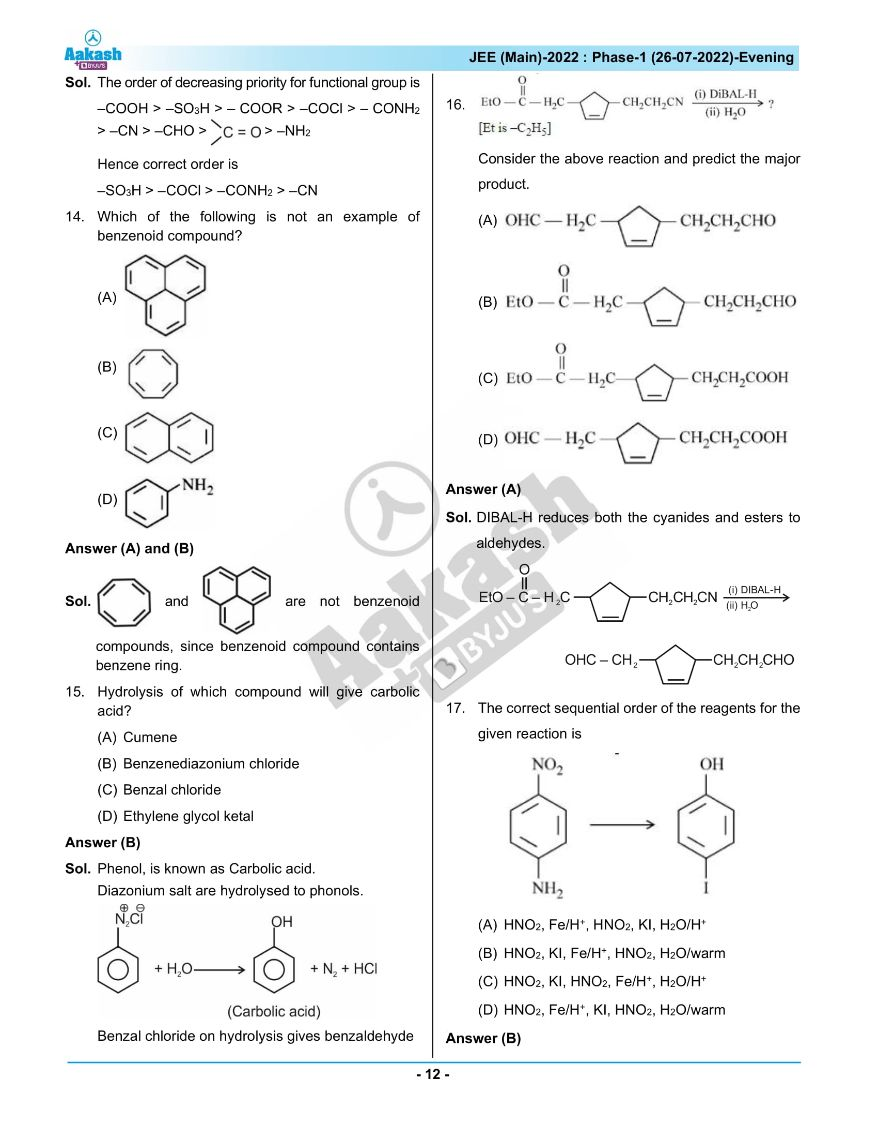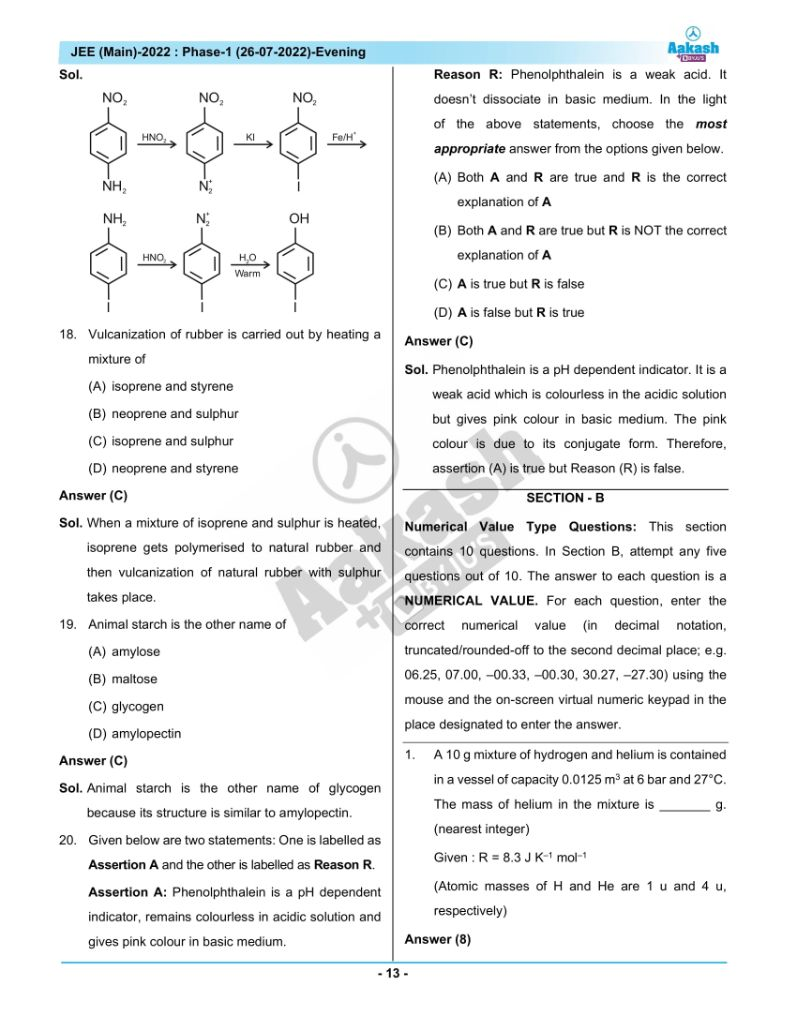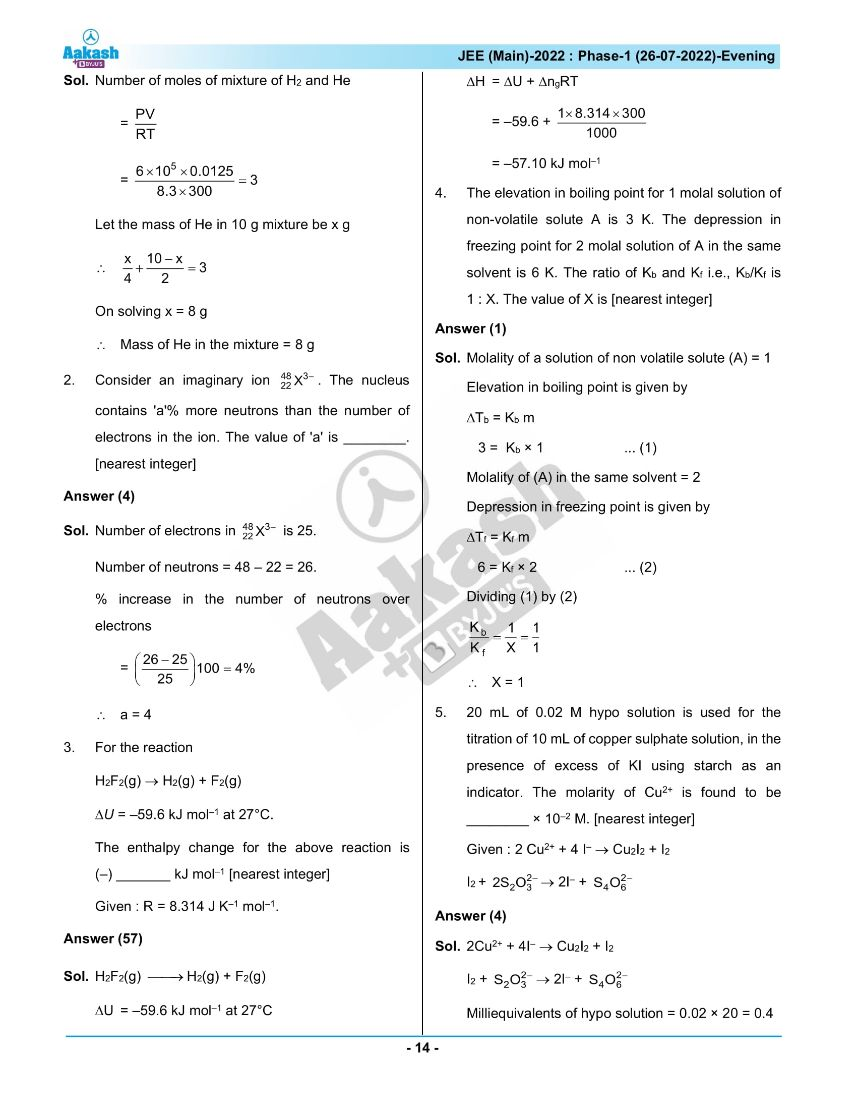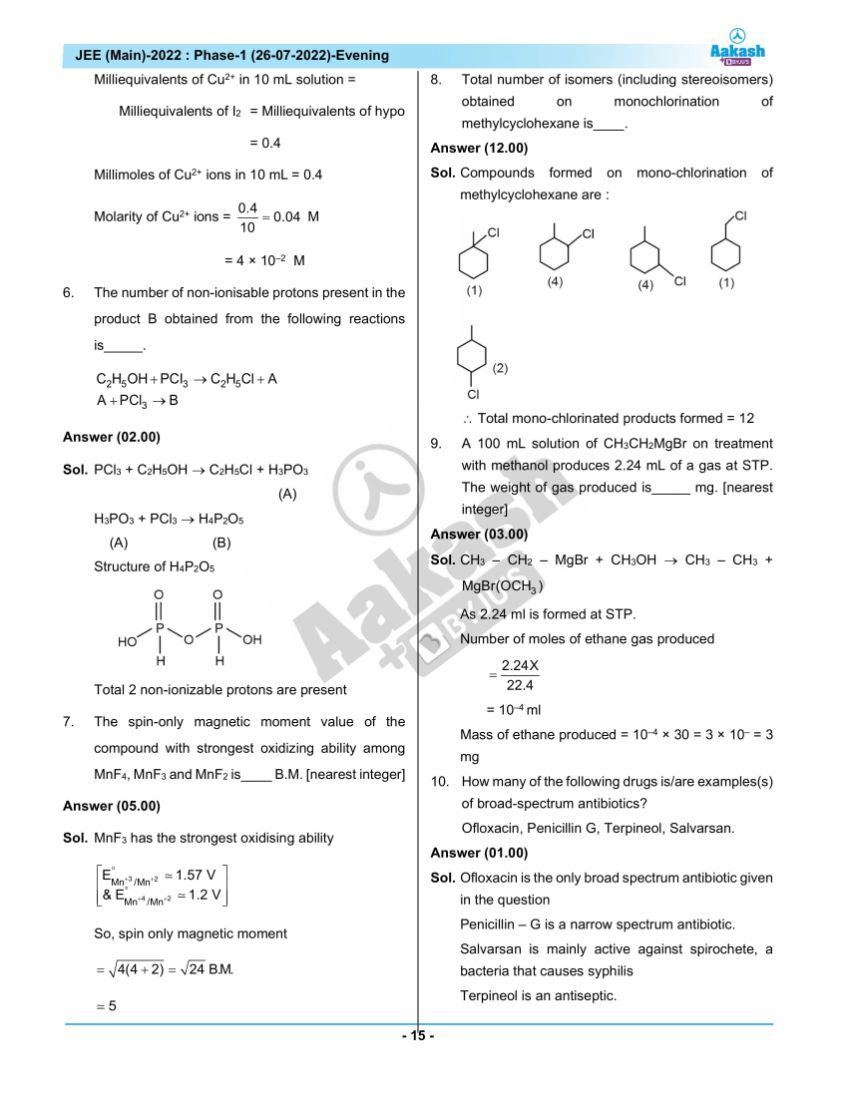## JEE Main 2022 July 26th Shift 2 Paper Analysis